Courses

# Test: Measurement - 2

## 20 Questions MCQ Test Mathematics (Maths) for Class 1 | Test: Measurement - 2

Description
This mock test of Test: Measurement - 2 for Class 1 helps you for every Class 1 entrance exam. This contains 20 Multiple Choice Questions for Class 1 Test: Measurement - 2 (mcq) to study with solutions a complete question bank. The solved questions answers in this Test: Measurement - 2 quiz give you a good mix of easy questions and tough questions. Class 1 students definitely take this Test: Measurement - 2 exercise for a better result in the exam. You can find other Test: Measurement - 2 extra questions, long questions & short questions for Class 1 on EduRev as well by searching above.
QUESTION: 1

Solution:
QUESTION: 2

Solution:
QUESTION: 3

### Look the picture below and answer the following question.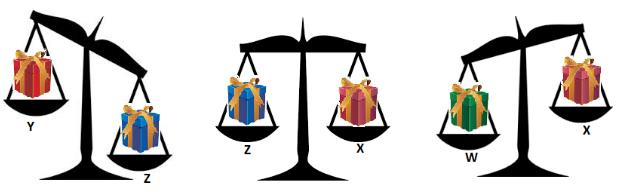The lightest box is ______.

Solution:
QUESTION: 4

Select the shorter

Solution:
QUESTION: 5

Choose the Taller

Solution:
QUESTION: 6

Rocha, Jian and Sasha have the following amount of money. Which two girls have the same amount of money?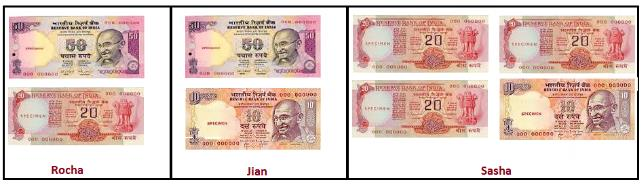_______ and _____ have the same amount of money.

Solution:
QUESTION: 7

Observe the figure and identify the distance the car travels, from the following options.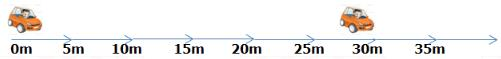Solution:
QUESTION: 8

Length measured upwards is _____.

Solution:
QUESTION: 9

Identify the shorter

Solution:
QUESTION: 10

Identify the thicker object

Solution:
QUESTION: 11

Pick out the thinner object

Solution:
QUESTION: 12

In the figure given below, who is closer to the finishing line?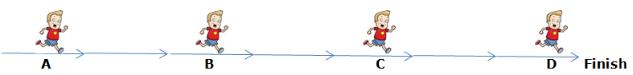Solution:
QUESTION: 13

Choose the heavier one

Solution:
QUESTION: 14

Anusha baked 5 cakes. The weight of each cake is 3 kg. What will be the total weight of 5 cakes?

Solution:

The weight of 1 cake is = 3 kg The weight of 5 cakes is = 3 + 3 + 3 + 3 + 3 = 15 kg.

QUESTION: 15

Select the lighter one

Solution:
QUESTION: 16

Which of the following houses are of the same height?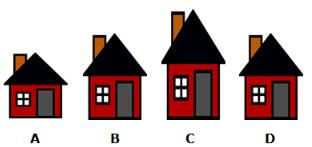Solution:
QUESTION: 17

Which of the following is the shortest?

Solution:
QUESTION: 18

Which container can hold more water than a bucket?

Solution:

Water tank can hold more water than a bucket.

QUESTION: 19

Identify the thickest one

Solution:
QUESTION: 20

Which object is thinner than your water bottle?

Solution:

Both pen and needle are thinner than bottle.